### Low drop-out linear regulators for low noise mobile electronics

Consumer demand seems to dictate a need for smaller and smaller electronics, thus driving designs toward down-scaling. Power efficiency, alloted area, user life, and maximum operating conditions are just some crucial elements factored in these designs. An example is the low-drop out regulator or LDO. It is a very simple and cheap low power source for mobile devices but isn't always the choice of designers due to its inefficiency when compared with switching regulators. The advantage it has over switching regulators is that it is less noisy and less expensive. Thus, LDOs find their place in a mobile device when a source with low noise is required (such as in wireless communications, where noise becomes a pivot of system reliability).

Rectification (turning AC to DC), voltage transformation (supplying the right DC level), filtering (removing frequency components from the rectified wave), regulation (maintaining the specified DC level), isolation (separating the main power source electrically to prevent surges and spikes from affecting the LDO), and protection (against overcurrents, overvoltages, ESD, and the like) are the key functions that any regulator must satisfy. Of particular interest is when LDOs are supplied with a voltage lower than their rated output. It is a matter worthy of argument since the real-life scenario for this is when the battery charge of the mobile device becomes very low. If the LDO were to shut down completely, and it were sourcing a significant amount of current to a still active load, it would shorten the life cycle, if not damage, the regulator.

Apparently, the structure of an LDO prevents such from occurring. Take the schematic of a basic LDO below.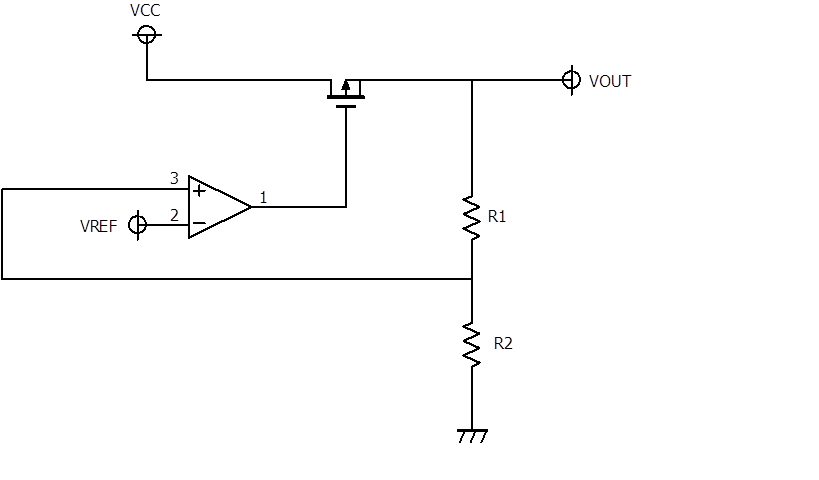Fig. 1 Schematic of a basic LDO employing a PMOS as a pass element.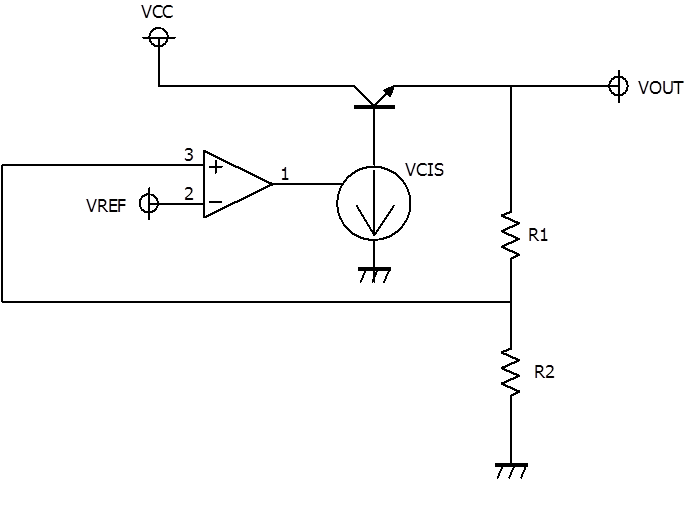Fig. 2 Schematic of a basic LDO employing a bipolar junction transistor as a pass element.

An LDO regulates its output through a feedback path. A sample of the output is compared to a reference voltage, possibly a bandgap circuit (a voltage reference designed to be unaffected by environmental factors). The difference is used to adjust the current (and consequently, the potential) appearing at the output. When the voltage at the input (Vcc) becomes lower than the rated output, the transistor becomes a short circuit to the input. The output of the regulator follows Vcc.

Now, what piqued my interest is figuring out the ability of the LDO to regulate given a switched load, when Vcc is lower than the rated Vout. When the regulator output is following the input, and a load pulse is applied, the transient characteristic measured does not represent the LDO at all. This is why some commercial ICs have trimmable LDO outputs. In order to properly evaluate the LDO, the output is "programmed" to a lower level. In this way, the feedback, decision-making, and regulating components of the LDO are still surely operating. (Trimming the output of commercial regulators is achieved by communicating with the IC via a standard, usually through an I2C interface).

It is also worth noting that application notes of LDOs have capacitors at their inputs and outputs. These capacitors are typically ceramic or tantalum capacitors which serve as a bypass for AC signals.

### From LDO Stability to simulation problems

Anyone who has taken a course on feedback systems would know that anything with feedback can oscillate, and the condition for oscillation can be deduced from the transfer function of the system.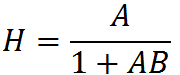And for oscillation to occur, the following must be satisfied.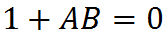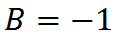For oscillation to occur, H approaches a very large number (infinity), the phase has to be inverted (i.e 180 deg. phase shift) and the gain must equal 1 (or 0 dB). The best way to estimate the tolerance of the a feedback system against oscillation is by taking its bode plot and calculating the gain and phase margins from the figures. The gain margin will be the amount of gain you can put into a feedback system until the 0 dB point intersects with the 180 deg. point of the phase plot. The phase margin will be the amount of phase you can put into a feedback system until the 180 deg. point intersects with the 0 dB point. I think a better way of looking at it is by keeping in mind the semantics of the word "margin". (Anyway, there is another easier and more straightforward method in determining whether a circuit will oscillate. It is called "Pease's principle" which concerns whamming the circuit with all sorts of square waves)

Now, it is possible to find oscillations when evaluating load transients of LDOs. When re-designing a circuit to remove those oscillations, there are 2 possible courses of action: re-design the circuit from scratch or simulate the transient response and AC characteristics of the circuit. In re-designing the circuit, you would want to provide a wider phase and gain margin. One can take the function of the circuit with respect to time and take the laplace transform, then make a bode plot. Complicated and tedious calculations can be skipped with MATLAB's support on symbolic processing. Define the voltage function of the circuit as a symbolic equation and take the laplace using the "laplace('<equaiton>')" command. Then define "s" with "zpk(<zeros>,<poles>,<gain>)" and route to the equation. Finally, use the "bode" and "nyquist" commands to check the AC characteristics (though I haven't tested if "freqz" can be used).

Re-designing the circuit is a very exhausting process, and is only done when simulation and modification fail to solve the problem. Circuit modification would be based on the trend of the bode plots. Sometimes, an exacting simulation is required to ensure that oscillation won't occur in a circuit. Finnicky specifications would yield hundreds or even thousands and thousands of simulation results.  On the side, circuit simulation implements mathematical models to arrive at solutions, and these mathematical models are equations solved via numerical methods such as Newton-Rhapson. Anyone who has taken a course on numerical methods knows that an initial guess fastens the convergence time. Thus, setting an initial guess for nodes in your circuit can fasten simulation time, and is particularly useful when you have thousands and thousands of simulation results. Sometimes, another party would want to simulate the circuit and would want the settings you used. However, giving the settings for the guesses that has been queued in your local grid can cause errors in the other party. Thus, it is helpful to delete these kind of settings before handing them over.

### The latest models of measuring instruments are not always better

Newer instruments on the market does not always imply improved accuracy and precision. I have tried measuring an output wave using a new version of a power source instrument. What happened? I got huge ringing problems on the high and low levels of my waveform. I first tried switching to an active probe, given that the capacitance of active probes are 6-9 pF lower than passive probes, in vain. Then I suspected the probing technique, then the environment, and finally the instruments. I tried switching to an older version of the instrument and... Eureka! the oscillation vanished into thin air. I tried the newer power source and the oscillation came back. Then I switched to the old one and it disappeared. I kept repeating the switch because I had a hard time accepting that an archaic instrument could still outperform a newer version in some ways. After a deeper investigation, it turned out that the older version had better voltage characteristics, BUT lower current stability and a measurement resolution lower than the newer version by a factor of 10.

### Statistical analysis and multivariate relationships

On a mathematical note, some statistical analysis methods can help aid in data interpretation. Variance and standard deviation determine how much a set of data such as a population or group of samples (note:samples use "N-1") are spread out/how far apart they are from each other. But STD and variance are only univariate. What if I want to compare 2 sets of data? What could quantify the similarity or difference between them? Then, multivariate statistics would come into play, such as covariance and correlation. Covariance takes the direction/trend of 2 data sets from each other (i.e. if one is increasing or decreasing from the other) while correlation takes the magnitude of similarity/difference between 2 data sets (i.e. how similar or different are the 2 data sets from each other?) Mathematically, covariance is: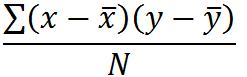while the correlation would simply be the covariance scaled down.

Conceptually, if (x-xmean) increases and (y-ymean) increases, then both data sets are increasing and their covariance will be large. However, if (x-xmean) increases while (y-ymean) decreases, the product will be a small number and the covariance will be small. Similarly, to properly compare the magnitudes or degree, both data sets will have to be scaled down by STDx and STDy.